## Introduction

Anaerobic digesters are broadly divided into batch and continuous types. Batch AD is a process in which all the substrates are supplied at the start, followed by product recovery at the end of the process. In contrast, feeding substrates are supplied and excreted daily in the same amount during the continuous AD process, resulting in a constant amount of digestate in the digester unlike that in batch AD. Generally, batch AD is performed to determine the maximum methane yield, calculate the hydraulic retention time, and determine various digestion characteristics for the setup of the control point before continuous AD operation.

Therefore, the present study aimed to evaluate the fermentation characteristics of bedded pack barn dairy cattle manure (BDCM), and to estimate the change in carbon and nitrogen content of its carbon fraction during batch SSAD.

## Materials & Methods

### Dairy cattle manure

The BDCM was collected at a facility with 0.02 head/m2 density of dairy cattle and 3.03 kg/m2 bedded sawdust in a cow farm in Yangpyung province (Latitude, 37.4614204; longitude, 127.5285745). The moisture content and volatile solids in sawdust were recorded as 36.1 ± 0.2% and 98.6 ± 0.1%, respectively. The BDCM characteristics considered in this study are listed in Table 1. Manure samples were randomly collected from at least five or more points and cow barns. Manure was collected eight times or more from each sampling point (Dou et al., 2001). The collected samples were classified using the VDI4630 method (Standard, 2006) and stored at −20 °C until further analysis.

The SSAD tests were carried out in triplicate using an approximately 1,400 mL polypropylene batch digester equipped with a 2 L gas bag at a constant temperature of 39 °C. The BDCM stored at 4 °C was pre-incubated at a temperature up to 39 °C before AD. The anaerobic state was established using N2 gas inside the bottles which were kept in a shaking incubator (IS-971R; Jeiotech Co., Korea) until less than 1% CH4 was produced. The substrate was supplied in the digester at 462.3 ± 4.9 g (wet basis) weight and moisture content adjusted to 82% using a mixture of distilled water, inoculum, and medium. Additionally, inoculum and medium were added at 0.88 and 0.44 g/g of total solids, respectively. The inoculum used in the study could digest cattle manure fed various substrates, and was collected from the batch type mesophilic anaerobic digester at 39 °C, which produced about 500 N mL CH4/g volatile solids (VSs)/d of biogas with 60% methane concentration The medium used for AD was prepared using the method described by Angelidaki & Sanders (2004) (Table 2). The final pH of the medium was adjusted to 7.1 using CO2 gas, and stored at 39 °C before AD. All assays were performed once every three days to measure gas production during the experimental period. Sampling was performed using a 50 mL gas-tight glass syringe (1001 SL SYR; Hamilton Co., Reno, USA) to measure gas production and composition every three days for 72 days. The sampling for recording pH, ammonia nitrogen, volatile fatty acids (VFA), and chemical composition was performed on the 0, 3, 6, 12, 18, 24, 30, 36, 48, 60, and 72nd days.

### Analytical method

Total and volatile solids were determined using the method described by APHA APH (2018). Elemental analyses of C, H, N, S, and O were performed using an elemental analyzer (EA 1110, CE Instruments, Italy), and pH was determined using a pH meter (Orion 420A+; Thermo Electron Co., Waltham, MA, USA). Ammonia nitrogen content was determined using the method described by (Rice, Baird & Eaton, 2017) and an ELISA reader (Synergy 2, Biotek Co., USA) according to Eq. (1) (Drosg, 2013). ${\mathrm{NH}}_{3}\left(\mathrm{mg}/\mathrm{L}\right)=\frac{{\mathrm{NH}}_{4}-\mathrm{N}}{1+1{0}^{\left(0.0925+2728.795/\mathrm{T}-\mathrm{pH}\right)}}$ T: 273.15 K + gas temperature (° C)

Gas production was determined daily using a 50 ml glass syringe. The amount of gas produced was calibrated to standard temperature and pressure (STP 0 °C, 1 atm) considering the temperature-dependent volume using the following calibration (Eq. (2)): $\mathrm{V}={\mathrm{V}}_{\text{at T}°C}×\frac{273}{\left(273+\mathrm{T}\right)}×\frac{\left(\mathrm{P}-{\mathrm{P}}_{\mathrm{W}}\right)}{760}$ where, V is the gas production at 0 °C and 1 atm, Vat TC isthe gas production at TC, T is the temperature at the time of the volume measurement, P is the pressure at the time of the volume measurement, and Pw is the saturated water vapor pressure at TC. The gas composition was determined by using gas chromatography (HP 6890; Hewlett-Packard Co., Palo Alto, CA, USA) with a thermal conductivity detector. The sample (0.2 ml) containing helium as the carrier gas was injected into the column at a temperature of 60 °C and flow rate of 1.5 ml/min. The sample gas concentration was calibrated using a standard gas mixture consisting of 40% CH4–60% CO2 and 60% CH4–40% CO2.

The amount of methane produced from the serum bottle was calibrated using the calibration equation (Eq. (3)) presented by Shin (2002): ${\mathrm{C}}_{{\mathrm{CH}}_{4}\left(\mathrm{R}\right)}={\mathrm{C}}_{{\mathrm{CH}}_{4}}×\frac{100}{\left({\mathrm{C}}_{{\mathrm{CH}}_{4}}+{\mathrm{C}}_{{\mathrm{CO}}_{2}}\right)}$ where, CCH4(R) is the calibrated methane concentration (%), CCH4 is the measured methane concentration (%), and CCO2 is the measured carbon dioxide concentration (%). The volatile solid reduction rate was determined by the equation described by Kim et al. (2018).

### Ultimate biodegradability and biomass removal

Total volatile solids (TVSs) consisted of biodegradable volatile solids (BVSs) and non-BVSs, and the TVS residue (TVSe) after degradation was calculated using Eqs. (4)(6). The ultimate biodegradability was calculated by plotting the ratio of TVSe and initial TVS before degradation (TVS0) on the Y-axis and reciprocal of the operating time (1/time) on the X-axis according to the method described by Kim et al. (2018). $\text{Biomass removal (BMR)}={\text{CH}}_{4}\phantom{\rule{0.25em}{0ex}}\text{weight}+{\text{CO}}_{2}\phantom{\rule{0.25em}{0ex}}\text{weight}$ $\mathrm{BMR}=\frac{{\mathrm{V}}_{0}×\left(\frac{16\mathrm{g}}{1\text{mole}}×\frac{{\mathrm{CH}}_{4}}{100}+\frac{44\mathrm{g}}{1\text{mole}}×\frac{{\mathrm{CO}}_{2}}{100}\right)}{\frac{22.413\mathrm{l}}{\text{mole}}}$ ${\text{TVS}}_{\mathrm{e}}={\text{TVS}}_{0}-\text{BMR}$

### Kinetic modeling

The daily methane yield rate from BDCM during SSAD was simulated using the modified Gaussian and Gomperz equations (Kim et al., 2018). This model describes the daily methane yield in batch AD assuming that the kinetic growth of microbes including methanogens follows a normal distribution during the process. The Gaussian equation is presented as Eq. (7). $y=a×exp\left[-0.5{\left(\frac{t-{t}_{0}}{b}\right)}^{2}\right]$

y = Methane production rate (N mL/g VS) at any time t

t = digestion time (Day)

a = Constant (N mL/g VS/day)

b = Constant (Day)

t0 = Maximal methane production rate day (Day)

The methane yield potential (P), maximum methane production (Rm; N mL/g VS), and lag phase (λ) were based on the volume and concentration of the biogas measured under the conditions of this experiment using the modified Gompertz equation (Kafle & Chen, 2016). $\mathrm{M}=\mathrm{P}×exp\left\{-exp\left[\frac{{R}_{m}×\mathrm{e}}{\mathrm{P}}\left(\lambda -\mathrm{t}\right)+1\right]\right\}$ M: Cumulative methane yield (N mL/g VS)

P: Methane yield potential (N mL/g VS)

Rm: Maximum methane production (N mL/g VS day)

λ: Lag phase

t: Day

### Statistical analysis

Data were analyzed using PROC MIXED of the SAS package program (SAS Inst. Inc., Cary, NC, USA) using retention time as a fixed model. Orthogonal polynomial contrasts were performed using the CONTRAST option to verify significance and polynomials were determined using SAS PROC IML. Statistical differences were considered significant at p < 0.05, and all averages were presented as least square means. Figures were created using GraphPad Prism 7.0 (GraphPad Software, San Diego, CA, USA).

## Results

### Methane production, kinetic modeling, and methane content

The methane production on daily and cumulative basis during batch SSAD, and from the kinetic model using a modified Gompertz curve are shown in Fig. 1 and Table 3, respectively. The methane production from BDCM during batch SSAD increased rapidly from day 12 onwards with maximum yield of 19.6 ± 0.8 N mL/g VS/day, whereas maximum methane production was observed at 24.0 ± 1.6 days. The R2 value of the Gaussian parameter using the modified Gompertz equation was 0.5992. Cumulative methane of BDCM was 142.5 ± 6.0 N mL/g VS at the end of SSAD. The maximum methane potential of BDCM was recorded as 134.7 ± 9.9 N mL/g VS according to the Gomperz curve and maximum methane production rate was 6.1 ± 0.4 N mL/g VS/day. The lag phase was 12.9 ± 1.1 days. The R2 value of the Gompertz parameter using the modified Gompertz equation was 0.9373. The methane content of BDCM in the batch SSAD is shown in Fig. 2 with a maximum value of 76.1 ± 7.3%. Results demonstrated that methane content increased rapidly until the 18th day, and it was maintained at 62.4 ± 4.8% until the end of the experiment. The R2 value of the trend line obtained using the sigmoidal curve fitting was 0.9054.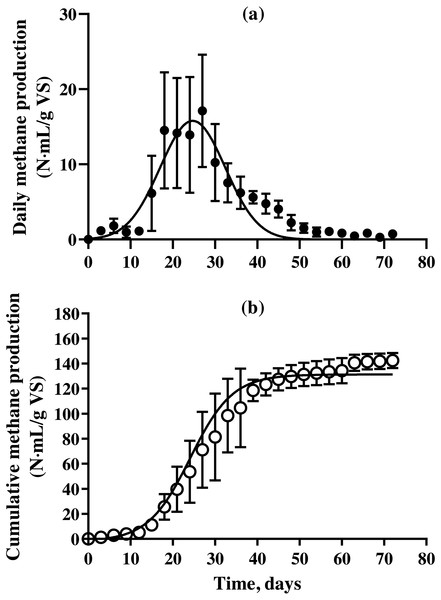Figure 1: Changes in the daily and cumulative methane production of bedded pack barn dairy cattle manure based on digestion time in the batch solid-state anaerobic digestion. Changes in the daily and cumulative methane production of bedded pack barn dairy cattle manure based on digestion time in the batch solid-state anaerobic digestion (n = 3) (Table S1A). Daily methane production (A, ○ ) and cumulative methane production (B, *). Error bars indicate standard deviation.   Download full-size image DOI: 10.7717/peerj.14134/fig-1

### Ultimate biodegradability (UB) and volatile solid removal rate

The UB and VS removal rates of BDCM in the batch SSAD were found to be 23.1 ± 3.4% and 19.9 ± 2.3, respectively (Table 4). Moreover, the BVS removal rate was 86.4 ± 1.4%.

### Fiber content removal

The results of cellulose, hemicellulose, and lignin contents of BDCM in SSAD are shown in Fig. 3. The cellulose and hemicellulose contents decreased linearly during SSAD (p = 0.001 and p < 0.001, respectively). In contrast, the lignin content increased (p = 0.001). The linear regression equations for cellulose, hemicellulose, and lignin content were as follows: cellulose (%, v. b.) = −0. 282 × fermentation time + 17.135, hemicellulose (%, v. b.) = −0. 629 × fermentation time + 16.126, and lignin (%, v. b.) = 0. 485 × fermentation time + 14.733.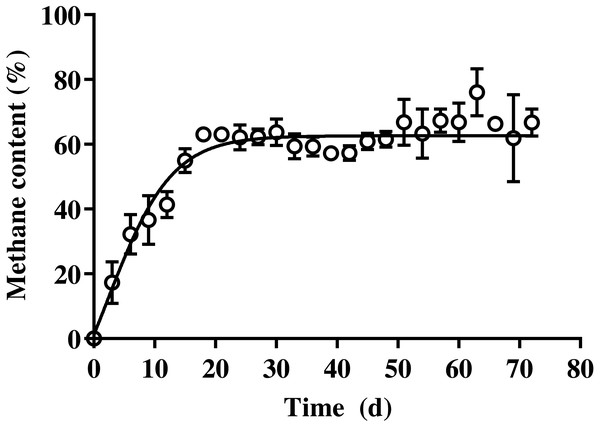Figure 2: Changes in the methane content of bedded pack barn dairy cattle manure based on digestion time in the batch solid-state anaerobic digestion. Changes in the methane content of bedded pack barn dairy cattle manure based on digestion time in the batch solid-state anaerobic digestion (n = 3) (Table S2A). Error bars indicate standard deviation.   Download full-size image DOI: 10.7717/peerj.14134/fig-2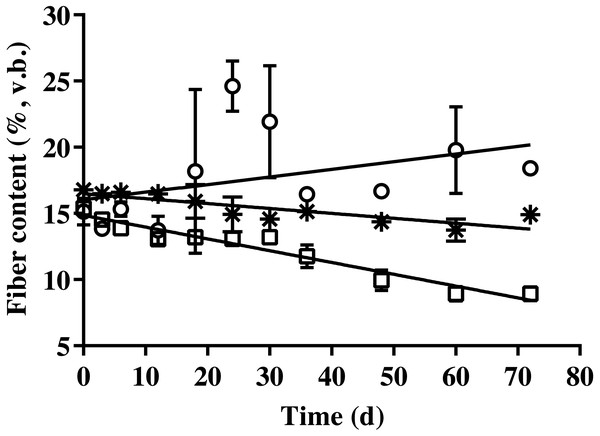Figure 3: Changes in the cellulose, hemicellulose, and lignin content of bedded pack barn dairy cattle manure according to digestion time in the batch solid-state anaerobic digestion. Changes in the cellulose, hemicellulose, and lignin content of bedded pack barn dairy cattle manure according to digestion time in the batch solid-state anaerobic digestion (n = 3) (Table S3A). Cellulose (*), hemicellulose (□), and lignin ( ○ ). v.b., Volatile solid basis. Error bars indicate standard deviation.   Download full-size image DOI: 10.7717/peerj.14134/fig-3

### Carbon and nitrogen removal

The carbon content of BDCM in the batch SSAD decreased quadratically during SSAD (p < 0.001; Fig. 4). The regression equations for non-fibrous carbon fraction (NFCF), hemicellulose carbon fraction (HCF), and acid detergent insoluble carbon (ADIC) were as follows: NFCF (%, v. b.) = 0.001 × Fermentation time2 - 0.1066 × fermentation time + 15.268, HCF (%, v. b.) = y = 0.0019 × Fermentation time2 - 0.2172 × fermentation time + 16.125, and ADIC (%, v. b.) = y = 0.0001 × fermentation time2- 0.0417 × fermentation time + 10.76.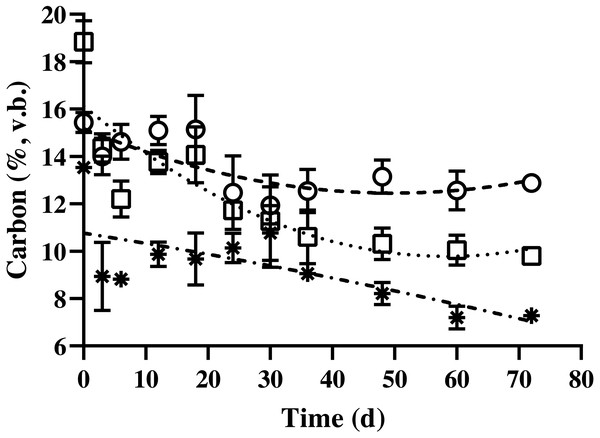Figure 4: Changes in the carbon content of bedded pack barn dairy cattle manure based on digestion time in the batch solid-state anaerobic digestion. Changes in the carbon content of bedded pack barn dairy cattle manure based on digestion time in the batch solid-state anaerobic digestion (n = 3) (Table S4A). ○ , Non-fibrous carbon fraction (quadratic equation, y = 0.001 × fermentation time2- 0.1066 × fermentation time + 15.268). □, Hemicellulose carbon fraction (quadratic equation, y = 0.0019 × fermentation time2- 0.2172 × fermentation time + 16.125); *, acid detergent insoluble carbon (cellulose + lignin; quadratic equation, y = 0.0001 × fermentation time2- 0.0417 × fermentation time + 10.76); v.b., volatile solid basis. Error bars indicate standard deviation.   Download full-size image DOI: 10.7717/peerj.14134/fig-4

The nitrogen content of BDCM in the batch SSAD is shown in Fig. 5. Results revealed that the non-fibrous nitrogen fraction (NFNF) and hemicellulose nitrogen fraction (HNF) contents decreased quadratically (p = 0.002 and p = 0.038, respectively). In contrast, no significant decrease in acid detergent insoluble nitrogen (ADIN) content was observed (p = 0.836). NFNF and HNF rapidly decreased until the day 12 and 6 of digestion, respectively (p < 0.001 and p = 0.008) but did not significantly decrease after day 15 until the end of the experiment. The regression equations of the NFNF, HNF, and ADIN were as follows: NFNF (%, v. b.) = 1.119 + (0.378/(1 + (Fermentation time/4.747)2.686), HNF (%, v. b.) = 0.268 + (0.431/(1 + (Fermentation time/2.737)19.421), and ADIN (%, v. b.) = 0.319 + (−0.046 / (1 + (Fermentation time/20.489)122.57)).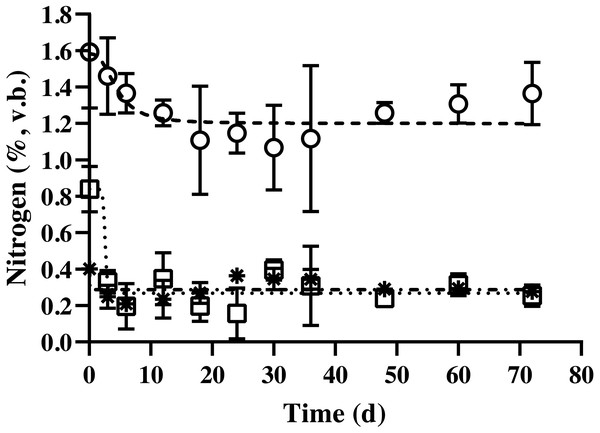Figure 5: Changes in the nitrogen content of bedded pack barn dairy cattle manure based on digestion time in the batch solid-state anaerobic digestion. Changes in the nitrogen content of bedded pack barn dairy cattle manure based on digestion time in the batch solid-state anaerobic digestion (n = 3) (Table S5A). ○ , Non-fibrous nitrogen fraction (Sysmetrical sigmoidal equation, y = 1.119 + (0.378/(1 + (Fermentation time/4.747)2.686); □, hemicellulose nitrogen fraction (Sysmetrical sigmoidal equation, y = 0.268 + (0.431/(1 + (Fermentation time/ 2.737)19.421); *, acid detergent insoluble nitrogen (cellulose + lignin; [Sysmetrical sigmoidal equation, y = 0.319 + (−0.046/(1 + (Fermentation time/20.489)122.57))]); %, volatile solid basis. Error bars indicate standard deviation.   Download full-size image DOI: 10.7717/peerj.14134/fig-5

### pH, NH3, NH4-N, and volatile fatty acids

Results demonstrated a change in the pH of BDCM ranging from 7.5–8.0 in batch SSAD until day 12 of fermentation. However, it increased to 8.5 and was maintained at 8.0 thereafter (Fig. 6).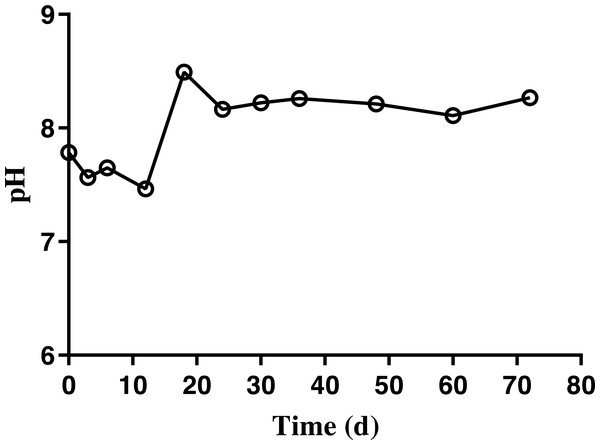Figure 6: Changes in the pH of bedded pack barn dairy cattle manure based on digestion time in the batch solid-state anaerobic digestion. Changes in the pH of bedded pack barn dairy cattle manure based on digestion time in the batch solid-state anaerobic digestion (n = 3). Error bars indicate standard deviation.   Download full-size image DOI: 10.7717/peerj.14134/fig-6

The ammonia nitrogen (NH4-N) content and maximum free ammonia (NH3) concentration of BDCM was maintained at 1,200 mg/L and 147.7 ± 13.8 mg/L during the entire batch SSAD (Fig. 7). However, the NH3 concentration decreased to <100 mg/L after 18th day.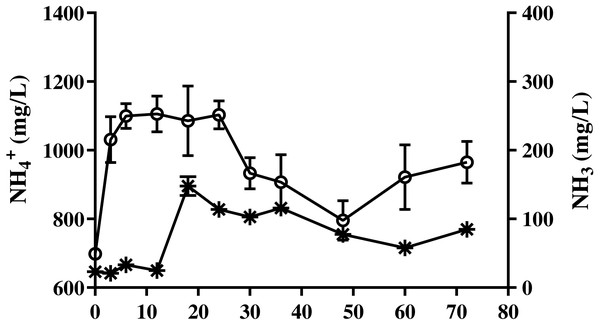Figure 7: Change in ammonia nitrogen and free ammonia content of bedded pack barn dairy cattle manure according to digestion time in batch solid-state anaerobic digestion. Change in ammonia nitrogen and free ammonia content of bedded pack barn dairy cattle manure according to digestion time in batch solid-state anaerobic digestion (n = 3). Ammonia nitrogen ( ○ , NH4-N), free ammonia (*, NH3). Error bars indicate standard deviation.   Download full-size image DOI: 10.7717/peerj.14134/fig-7

Results of VFA concentration of BDCM in the SSAD batch are shown in Fig. 8, and revealed that the maximum acetic, propionic, and VFA concentrations were 223.0 ± 10.8, 110.5 ± 11.9, and 357.3 ± 21.5 mg/L, respectively. Moreover, VFA concentrations were mostly maintained after 36 days of batch SSAD.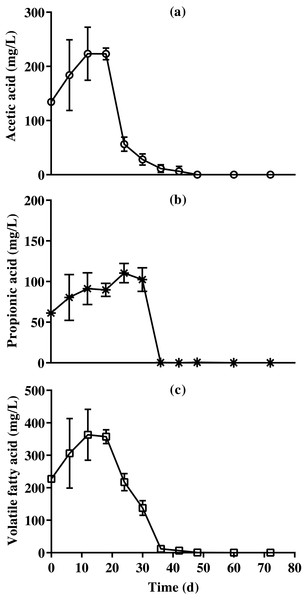Figure 8: Changes in acetic acid, propionic acid , and total volatile fatty acid content reduction of bedded pack barn dairy cattle manure based on the batch solid-state anaerobic digestion. Changes in acetic acid, propionic acid , and total volatile fatty acid content reduction of bedded pack barn dairy cattle manure based on the batch solid-state anaerobic digestion (n = 3). Acetic acid (A, ○ ), propionic acid (B, *), and total volatile fatty acid (C, □). Error bars indicate standard deviation.   Download full-size image DOI: 10.7717/peerj.14134/fig-8

## Discussion

The cumulative methane production of BDCM in the batch SSAD was 127.7 N mL/g VS after 45 days of fermentation, with a methane potential of 95.0% according to the kinetic model. This observation differed from previous reports (Triolo et al., 2011; Kim et al., 2018). Additionally, the average methane content was found to be 62.4% from day 18 to 72. However, according to previous studies, it ranges from 65 to 80% (Rosas-Mendoza et al., 2021, p.), or 40 to 55% during AD process (Wang et al., 2012; Goux et al., 2016). Further, our results demonstrated no change in the methane content of BDCM in the batch SSAD after a digestion time of 18–72 days. Moreover, no reduction in methane content also suggested that methane production was not inhibited during the AD process. According to a previous study (Triolo et al., 2011), cattle manure with similar contents of fibrous materials as used in our study, was able to produce 197–223.6 N mL/g VS of methane. Although the present study used similar substrates during SSAD, the moisture conditions, lignin content of feeding substrates, and substrate to inoculum ratio were different. The moisture conditions in the previous study were 89.6 and 92.5%, whereas our study reported it as 82%. Water activity is known to affect the microbial growth during the digestion process, and the optimum water activities may vary among microbial species (Gervais, Marechal & Molin, 1996). Methane production was the highest at 85% moisture in our study, and higher production occurred at 82% moisture than at 89.6 and 92.5% (Veluchamy & Kalamdhad, 2017). These findings suggest that moisture condition was not a factor associated with decreased methane production in the present study. Furthermore, our results reported lignin content of feeding substrate as 17.5% of dry matter (DM); however, previous studies have reported it as 29.1% (Kim et al., 2018) and 6.49–11.91% of DM (Triolo et al., 2011). Correspondingly, the methane production was 142.5 (our study), 90.3 (Kim et al., 2018), and 197.0–223.6 N mL g/g VS (Triolo et al., 2011). Increasing lignin content in feeding substrates causes a linear decrease in methane production and biochemical methane potential (BMP) (Triolo et al., 2011; Choi et al., 2020). Lignin is the primary component of recalcitrant fraction of lignocellulose (Khan & Ahring, 2019), and its biological conversion is limited in the absence of oxygen; however, anaerobic conditions are necessary for methane production by biological digestion (Harun et al., 2010). Additionally, sawdust is a by-product of woodworking such as sawing, sanding, routing, etc., and lignin constitutes 81.59% of VS of the pinewood sawdust (Muley et al., 2016). When the VS content was calculated excluding lignin content of substrates, it was found to be 85.4–88.4 (Triolo et al., 2011), 70.9 (Kim et al., 2018), and 85.5% of VS (our study). Therefore, the lignin content of substrates was a significant factor affecting methane production. Nevertheless, a huge difference in methane production was reported between the present study (142.5 N mL g/g VS) and that of Triolo et al. (2011) (197.0–223.6 N mL g/g VS). The substrate to inoculum (S/I) ratio has also been reported to affect the cumulative methane production which was the highest at S/I ratio 1.0; however, it was found to be lower (approximately half) at other S/I ratios (0.25, 1.5, and 2.0) (Haider et al., 2015). The study by Triolo et al. (2011) was conducted at S/I ratio of 1.0 while we performed it with 0.05. Therefore, the difference observed in cumulative methane production may have resulted from varying S/I ratios. The UB and VS removal rates in our study were found to be lower than those reported in previous studies (Khalid et al., 2011). Furthermore, as UB and VS removal rates are calculated using methane and carbon dioxide yields, the lower values may be a result of low methane yield.

The equation of fibrous contents is presented to evaluate the degradation of each fibrous fraction. Results revealed that the hemicellulose and cellulose contents of BDCM decreased during SSAD, while the lignin content increased. Unmodified lignin is an undegradable component of the AD process (Khan & Ahring, 2019). In this study, the cellulose content was calculated by subtracting the lignin from acid detergent fiber content, and the hemicellulose content was calculated by subtracting the acid detergent fiber from the neutral detergent fiber content. Therefore, increase in lignin content with fermentation progress may have resulted from the decomposition of cellulose and hemicellulose. In addition, the fibrous content showed that cellulose degraded more slowly than hemicellulose (Fig. 3). Previous reports showed that xylan, which is hemicellulose, degrades faster than starch and cellulose during the BMP test (Choi, Ryu & Lee, 2020). Our study also demonstrated the same results. Therefore, hemicellulose is considered to be the most rapid degradable fraction of fibrous materials during SSAD.

Although a pH of over 8.0 was reported that inhibited the AD (Cioabla et al., 2012), no inhibition due to pH change was observed during the entire batch SSAD process. In AD process, the inhibition of ammonia nitrogen (NH4-N) and free ammonia (NH3) was known to occur at over 3,000 and 80 mg/L, respectively (Yenigün & Demirel, 2013). In our study, NH4-N content was under 1,200 mg/L. Serious inhibition has been reported at a pH of 8.0 and at over 100 mg/L of NH3 in the AD process; however, no inhibition possibly occurred in this study as the NH3 concentration was under 100 mg/L after 18 days. The inhibition by VFA accumulation has been reported to occur at over 2,400 (Wang et al., 2009), 2,750, (Drosg, 2013), and 4,000 mg/L (Lee et al., 2015). The results of this study suggest that the VFA content does not inhibit the entire SSAD process.

Overall, the BDCM used in this study was found to have low biodegradability, owing to the incorporation of sawdust. In addition, digestion proceeded normally without any inhibitory action during the entire SSAD process. Further, differences in carbohydrate structure of the digested material in the batch SSAD and ADIN were confirmed to make degradation difficult during the AD process. Therefore, this study provides information on BDCM digestion characteristics in the SSAD which can be of significant help in improving the SSAD process.

## Supplemental Information

### Competing Interests

The authors declare there are no competing interests. Youngjun Na is an employer (chief executive officer) of Animal Data Lab., Antller Inc.

### Author Contributions

Yongjun Choi conceived and designed the experiments, performed the experiments, analyzed the data, prepared figures and/or tables, authored or reviewed drafts of the article, and approved the final draft.

Sangrak Lee conceived and designed the experiments, prepared figures and/or tables, authored or reviewed drafts of the article, and approved the final draft.

Duck-Min Ha performed the experiments, prepared figures and/or tables, and approved the final draft.

Youngjun Na analyzed the data, authored or reviewed drafts of the article, and approved the final draft.

Doo-Hwan Kim conceived and designed the experiments, authored or reviewed drafts of the article, and approved the final draft.

### Data Availability

The following information was supplied regarding data availability:

All raw data are available in the Supplementary Files.

### Funding

This research was supported by the Basic Science Research Program through the National Research Foundation of Korea (NRF) funded by the Ministry of Education (2021R1I1A1A01058751) and the 2018 Post-Doc Department of Animal Resources Technology, Gyeongnam National University of Science and Technology, and Agri-Food Bio Convergence Institute. The funders had no role in study design, data collection and analysis, decision to publish, or preparation of the manuscript.